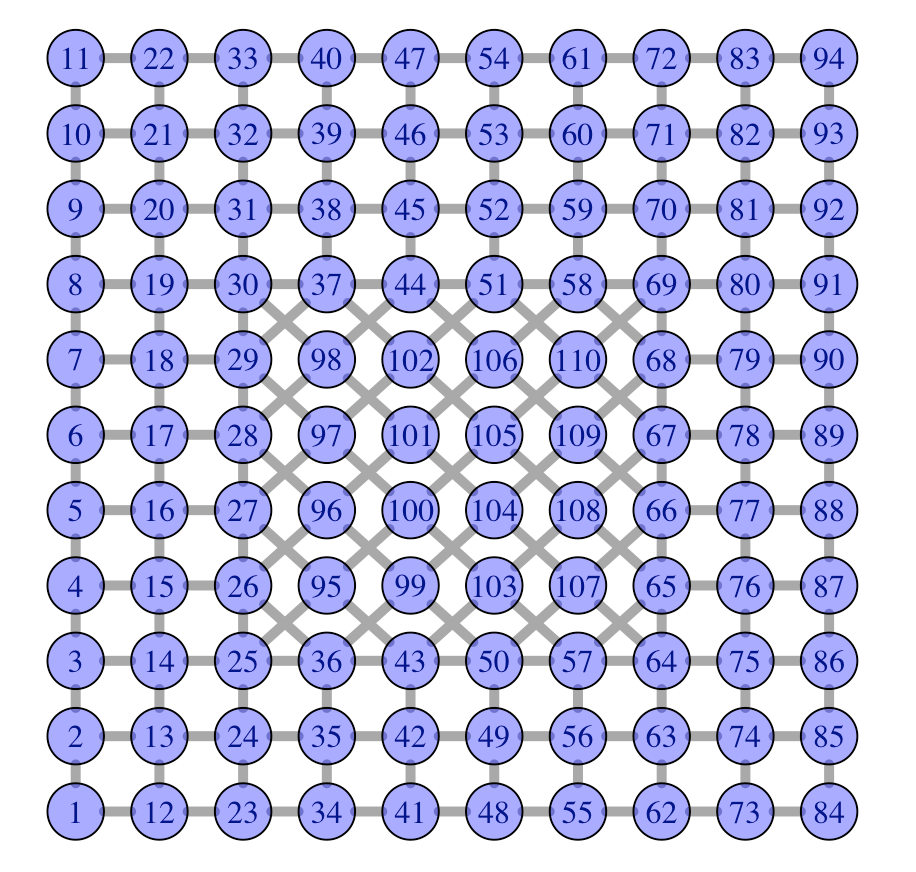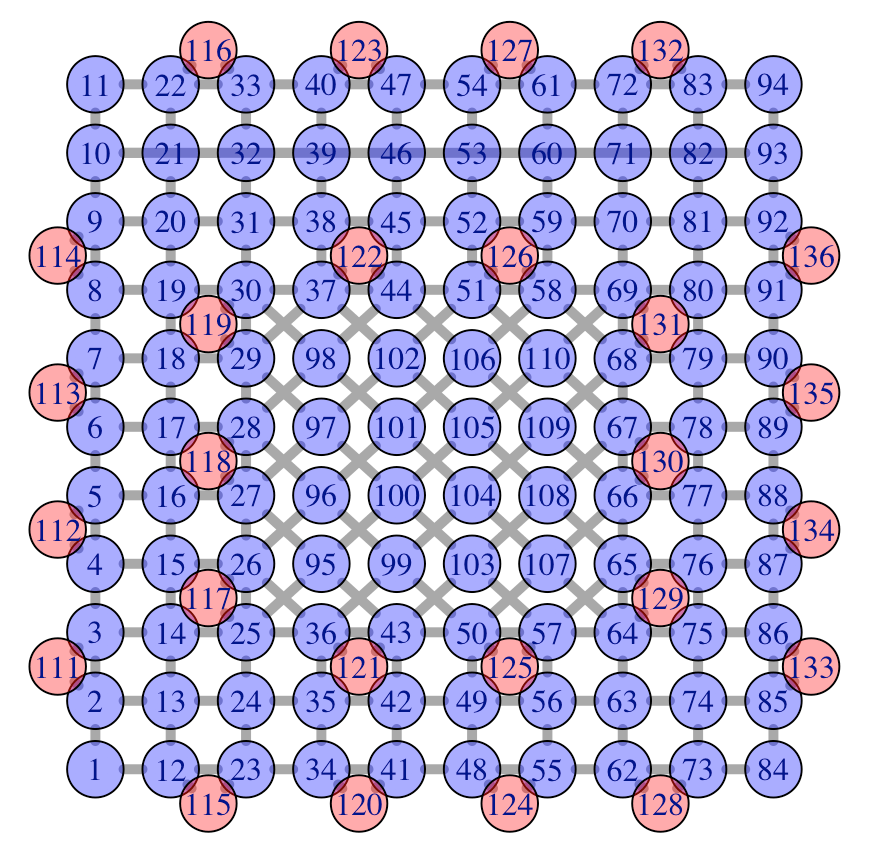# Solving the chinese postman problem

October 19, 2018
By

Want to share your content on R-bloggers? click here if you have a blog, or here if you don't.

Some pre-Halloween post today. It started actually while I was in Barcelona : kids wanted to go back to some store we’ve seen the first day, in the gothic part, and I could not remember where it was. And I said to myself that would be quite long to do all the street of the neighborhood. And I discovered that it was actually an old problem. In 1962, Meigu Guan was interested in a postman delivering mail to a number of streets such that the total distance walked by the postman was as short as possible. How could the postman ensure that the distance walked was a minimum?

A very close notion is the concept of traversable graph, which is one that can be drawn without taking a pen from the paper and without retracing the same edge. In such a case the graph is said to have an Eulerian trail (yes, from Euler’s bridges problem). An Eulerian trail uses all the edges of a graph. For a graph to be Eulerian all the vertices must be of even order.

An algorithm for finding an optimal Chinese postman route is:

1. List all odd vertices.
2. List all possible pairings of odd vertices.
3. For each pairing find the edges that connect the vertices with the minimum weight.
4. Find the pairings such that the sum of the weights is minimised.
5. On the original graph add the edges that have been found in Step 4.
6. The length of an optimal Chinese postman route is the sum of all the edges added to the total found in Step 4.
7. A route corresponding to this minimum weight can then be easily found.

For the first steps, we can use the codes from Hurley & Oldford’s Eulerian tour algorithms for data visualization and the PairViz package. First, we have to load some R packages

 ```1 2 3 4 ``` ```require(igraph) require(graph) require(eulerian) require(GA)```

Then use the following function from stackoverflow,

 ```1 2 3 4 5 6 7 8 9 10 11 12 13 14 15 16 17 18 19 20 21 22 23 24 25 26 27 28 29 30 31 32 33 34 ``` ```make_eulerian = function(graph){ info = c("broken" = FALSE, "Added" = 0, "Successfull" = TRUE) is.even = function(x){ x %% 2 == 0 } search.for.even.neighbor = !is.even(sum(!is.even(degree(graph)))) for(i in V(graph)){ set.j = NULL uneven.neighbors = !is.even(degree(graph, neighbors(graph,i))) if(!is.even(degree(graph,i))){ if(sum(uneven.neighbors) == 0){ if(sum(!is.even(degree(graph))) > 0){ info["Broken"] = TRUE uneven.candidates <- !is.even(degree(graph, V(graph))) if(sum(uneven.candidates) != 0){ set.j <- V(graph)[uneven.candidates][] }else{ info["Successfull"] <- FALSE } } }else{ set.j <- neighbors(graph, i)[uneven.neighbors][] } }else if(search.for.even.neighbor == TRUE & is.null(set.j)){ info["Added"] <- info["Added"] + 1 set.j <- neighbors(graph, i)[ !uneven.neighbors ][] if(!is.null(set.j)){search.for.even.neighbor <- FALSE} } if(!is.null(set.j)){ if(i != set.j){ graph <- add_edges(graph, edges=c(i, set.j)) info["Added"] <- info["Added"] + 1 } } } (list("graph" = graph, "info" = info))}```

Then, consider some network, with 12 nodes

 ```1 2 3 ``` ```g1 = graph(c(1,2, 1,3, 2,4, 2,5, 1,5, 3,5, 4,7, 5,7, 5,8, 3,6, 6,8, 6,9, 9,11, 8,11, 8,10, 8,12, 7,10, 10,12, 11,12), directed = FALSE)```

To plot that network, use

 ```1 2 3 4 ``` ```V(g1)\$name=LETTERS[1:12] V(g1)\$color=rgb(0,0,1,.4) ly=layout.kamada.kawai(g1) plot(g1,vertex.color=V(newg)\$color,layout=ly)```Then we convert it to some traversable graph by adding 5 vertices

 ```1 2 3 4 5 ``` ```eulerian = make_eulerian(g1) eulerian\$info broken Added Successfull 0 5 1 g = eulerian\$graph```

as shown below

 ```1 2 ``` ```ly=layout.kamada.kawai(g) plot(g,vertex.color=V(newg)\$color,layout=ly)```We cut those 5 vertices in two part, and therefore, we add 5 artificial nodes

 ```1 2 3 4 5 6 7 8 9 10 11 12 13 14 15 ``` ```A=as.matrix(as_adj(g)) A1=as.matrix(as_adj(g1)) newA=lower.tri(A, diag = FALSE)*A1+upper.tri(A, diag = FALSE)*A for(i in 1:sum(newA==2)) newA = cbind(newA,0) for(i in 1:sum(newA==2)) newA = rbind(newA,0) s=nrow(A) for(i in 1:nrow(A)){ Aj=which(newA[i,]==2) if(!is.null(Aj)){ for(j in Aj){ newA[i,s+1]=newA[s+1,i]=1 newA[j,s+1]=newA[s+1,j]=1 newA[i,j]=1 s=s+1 }}}```

We get the following graph, where all nodes have an even number of vertices !

 ```1 2 3 4 5 6 7 8 9 10 11 12 ``` ```newg=graph_from_adjacency_matrix(newA) newg=as.undirected(newg) V(newg)\$name=LETTERS[1:17] V(newg)\$color=c(rep(rgb(0,0,1,.4),12),rep(rgb(1,0,0,.4),5)) ly2=ly transl=cbind(c(0,0,0,.2,0),c(.2,-.2,-.2,0,-.2)) for(i in 13:17){ j=which(newA[i,]>0) lc=ly[j,] ly2=rbind(ly2,apply(lc,2,mean)+transl[i-12,]) } plot(newg,layout=ly2)```Our network is now the following (new nodes are small because actually, they don’t really matter, it’s just for computational reasons)

 ```1 2 3 ``` ```plot(newg,vertex.color=V(newg)\$color,layout=ly2, vertex.size=c(rep(20,12),rep(0,5)), vertex.label.cex=c(rep(1,12),rep(.1,5)))```Now we can get the optimal path

 ```1 2 3 4 5 6 7 ``` ```n <- LETTERS[1:nrow(newA)] g_2 <- new("graphNEL",nodes=n) for(i in 1:nrow(newA)){ for(j in which(newA[i,]>0)){ g_2 <- addEdge(n[i],n[j],g_2,1) }} etour(g_2,weighted=FALSE)  "A" "B" "D" "G" "E" "A" "C" "E" "H" "F" "I" "K" "H" "J" "G" "P" "J" "L" "K" "Q" "L" "H" "O" "F" "C"  "N" "E" "B" "M" "A"```

or

 ```1 2 3 4 5 6 7 8 9 10 11 12 13 14 ``` ```edg=attr(E(newg), "vnames") ET=etour(g_2,weighted=FALSE) parcours=trajet=rep(NA,length(ET)-1) for(i in 1:length(parcours)){ u=c(ET[i],ET[i+1]) ou=order(u) parcours[i]=paste(u[ou],u[ou],sep="|") trajet[i]=which(edg==parcours[i]) } parcours  "A|B" "B|D" "D|G" "E|G" "A|E" "A|C" "C|E" "E|H" "F|H" "F|I" "I|K" "H|K" "H|J" "G|J" "G|P" "J|P"  "J|L" "K|L" "K|Q" "L|Q" "H|L" "H|O" "F|O" "C|F" "C|N" "E|N" "B|E" "B|M" "A|M" trajet  1 3 8 9 4 2 6 10 11 12 16 15 14 13 26 27 18 19 28 29 17 25 24 7 22 23 5 21 20```Let us try now on a real network of streets. Like Missoula, Montana.I will not try to get the shapefile of the city, I will just try to replicate the photography above.If you look carefully, you will see some problem : 10 and 93 have an odd number of vertices (3 here), so one strategy is to connect them (which explains the grey line).But actually, to be more realistic, we start in 93, and we end in 10. Here is the optimal (shortest) path which goes through all vertices.Now, we are ready for Halloween, to go through all streets in the neighborhood !

R-bloggers.com offers daily e-mail updates about R news and tutorials about learning R and many other topics. Click here if you're looking to post or find an R/data-science job.
Want to share your content on R-bloggers? click here if you have a blog, or here if you don't.